# RD Sharma Solutions for Class 10 Maths Chapter 8 Quadratic Equations Exercise 8.4

The second method of solving quadratic equations is discussed in this exercise. Its called completing the square method. Not every student can grasp this method quickly. That’s why BYJU’S has created the RD Sharma Solutions Class 10 by subject experts to make students understand concepts easily and also clarify their doubts simultaneously. Students can also make use of the RD Sharma Solutions for Class 10 Maths Chapter 8 Quadratic Equations Exercise 8.4 PDF provided below.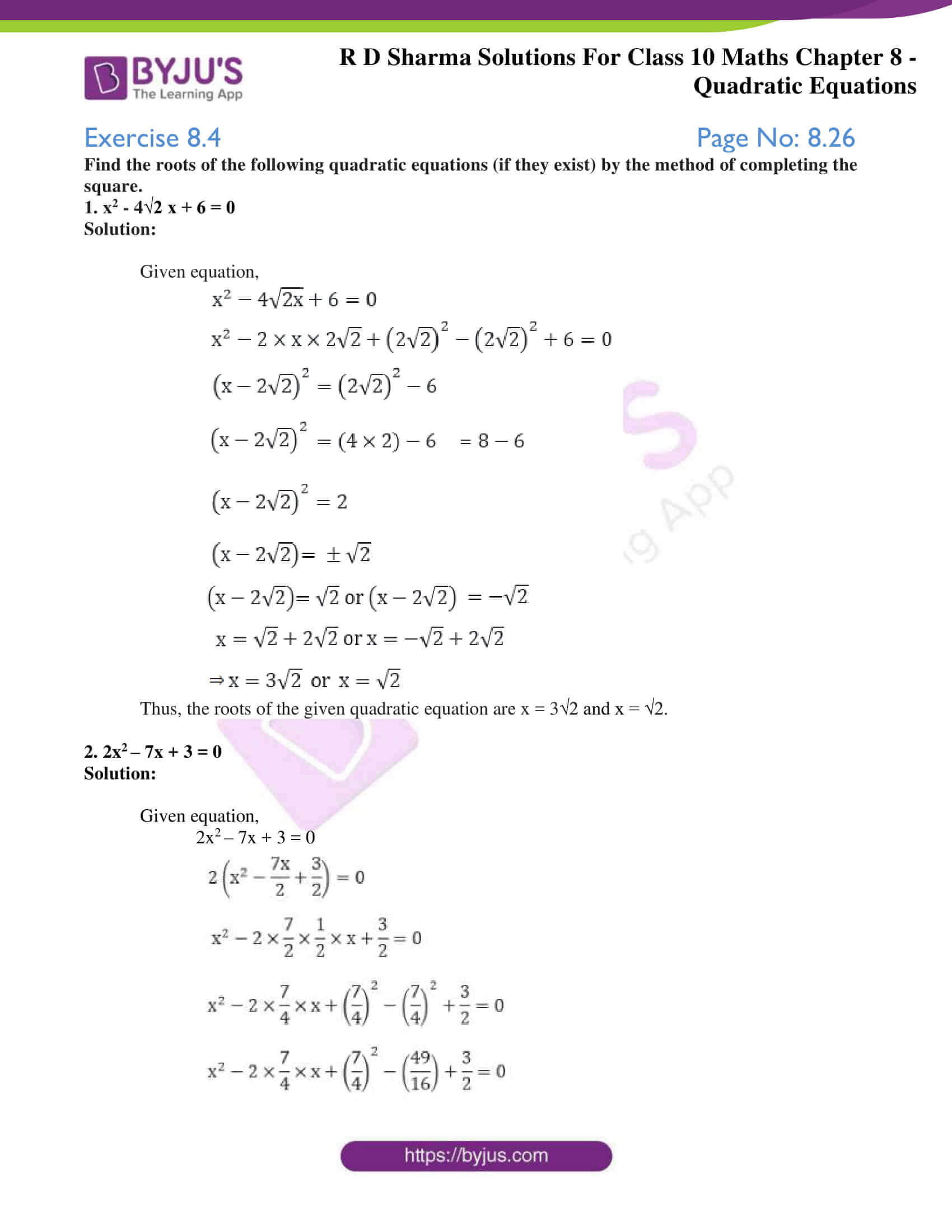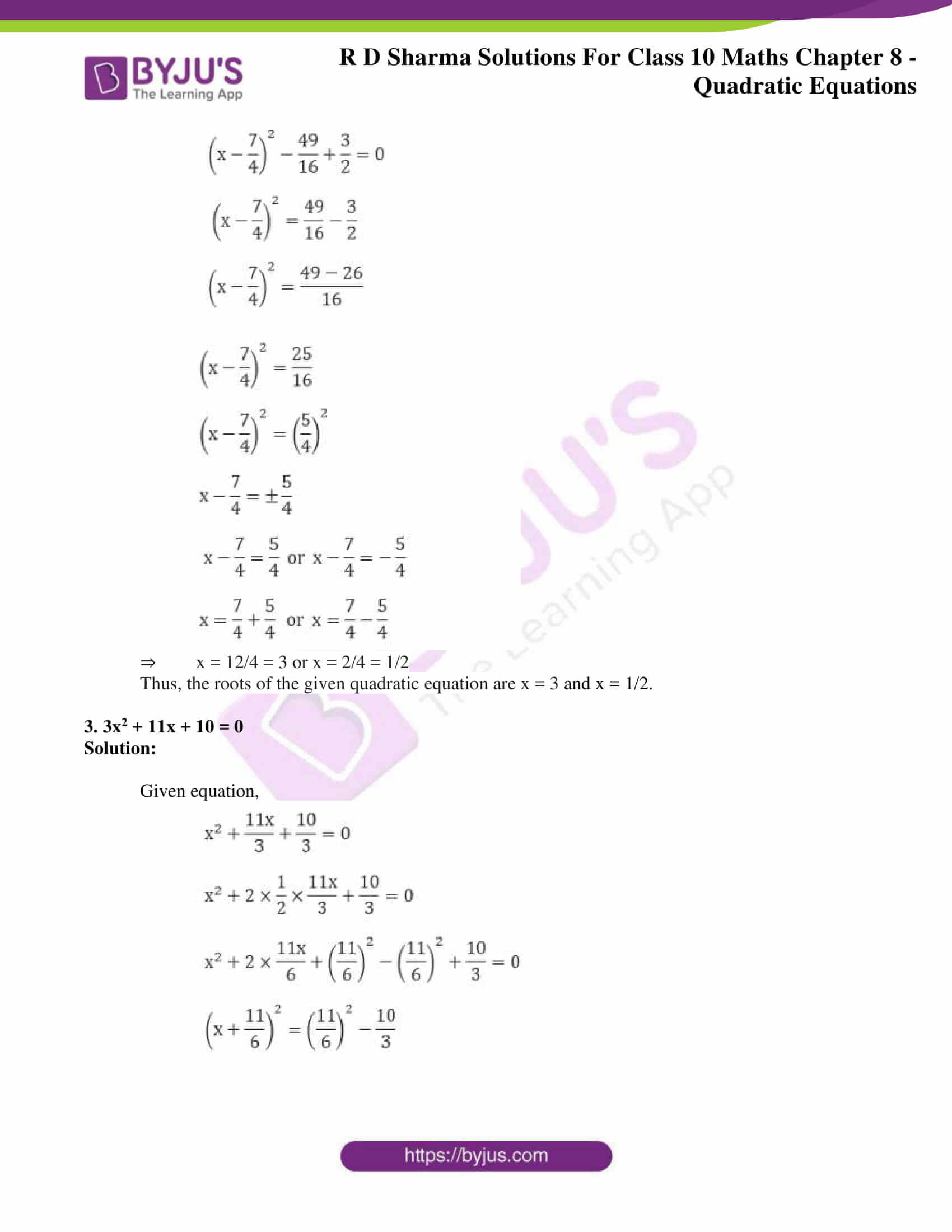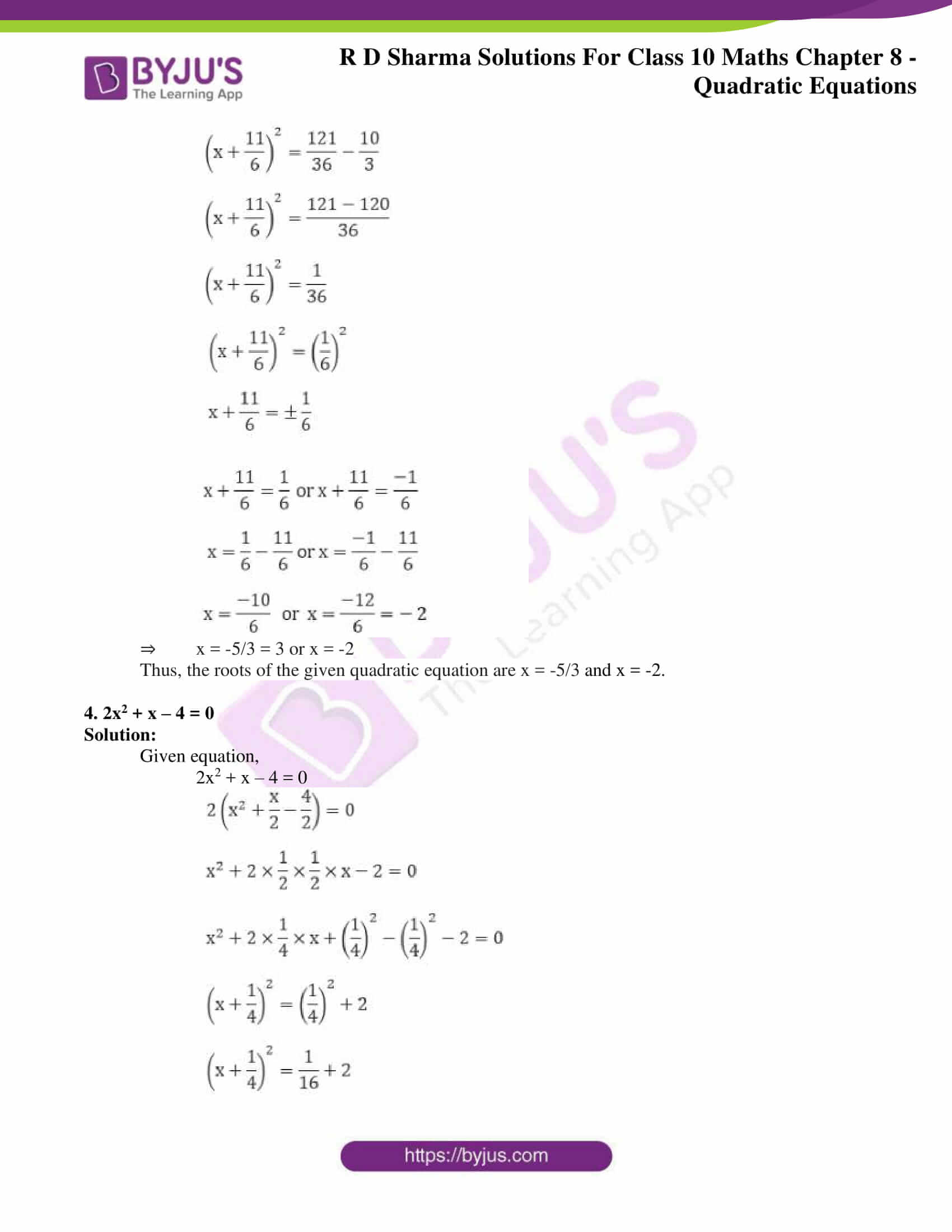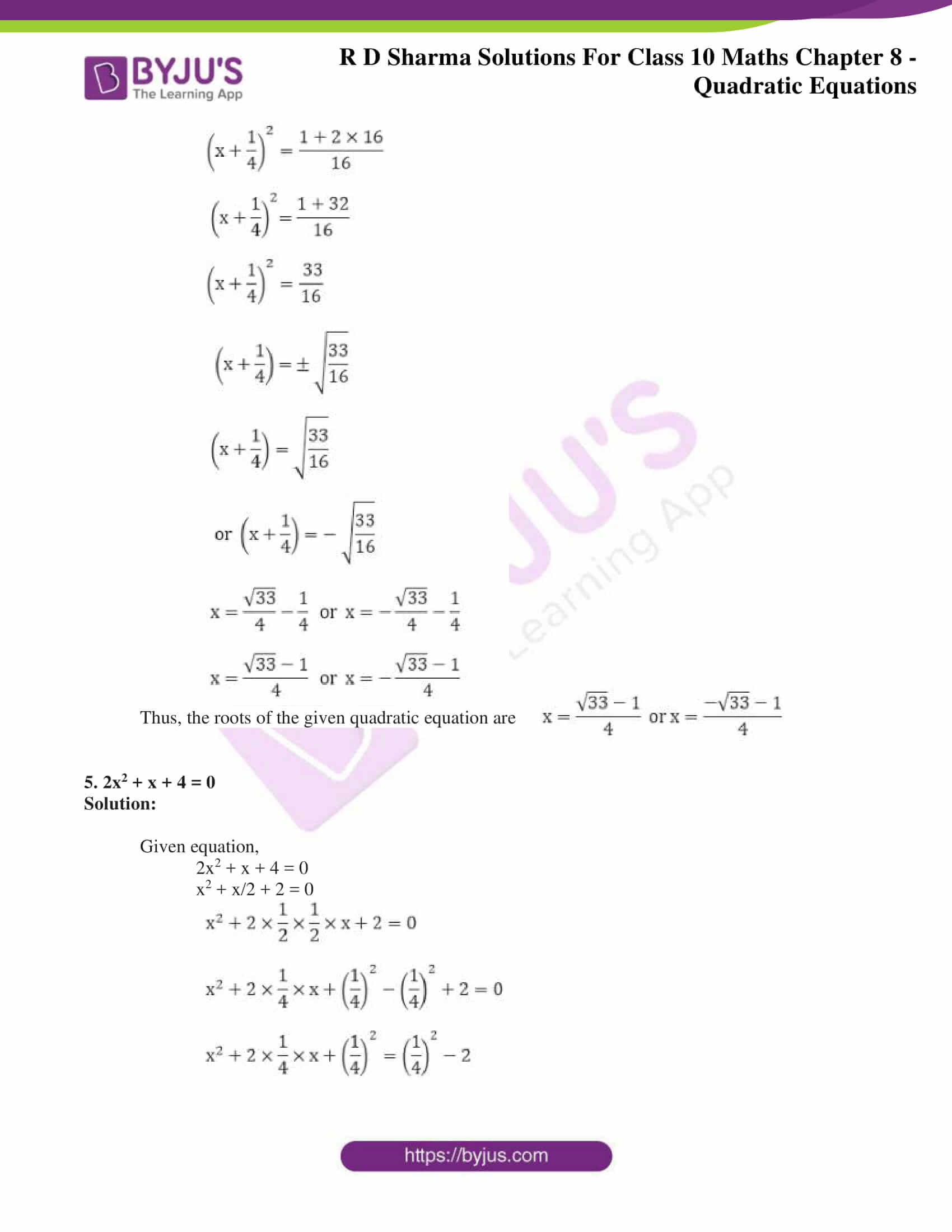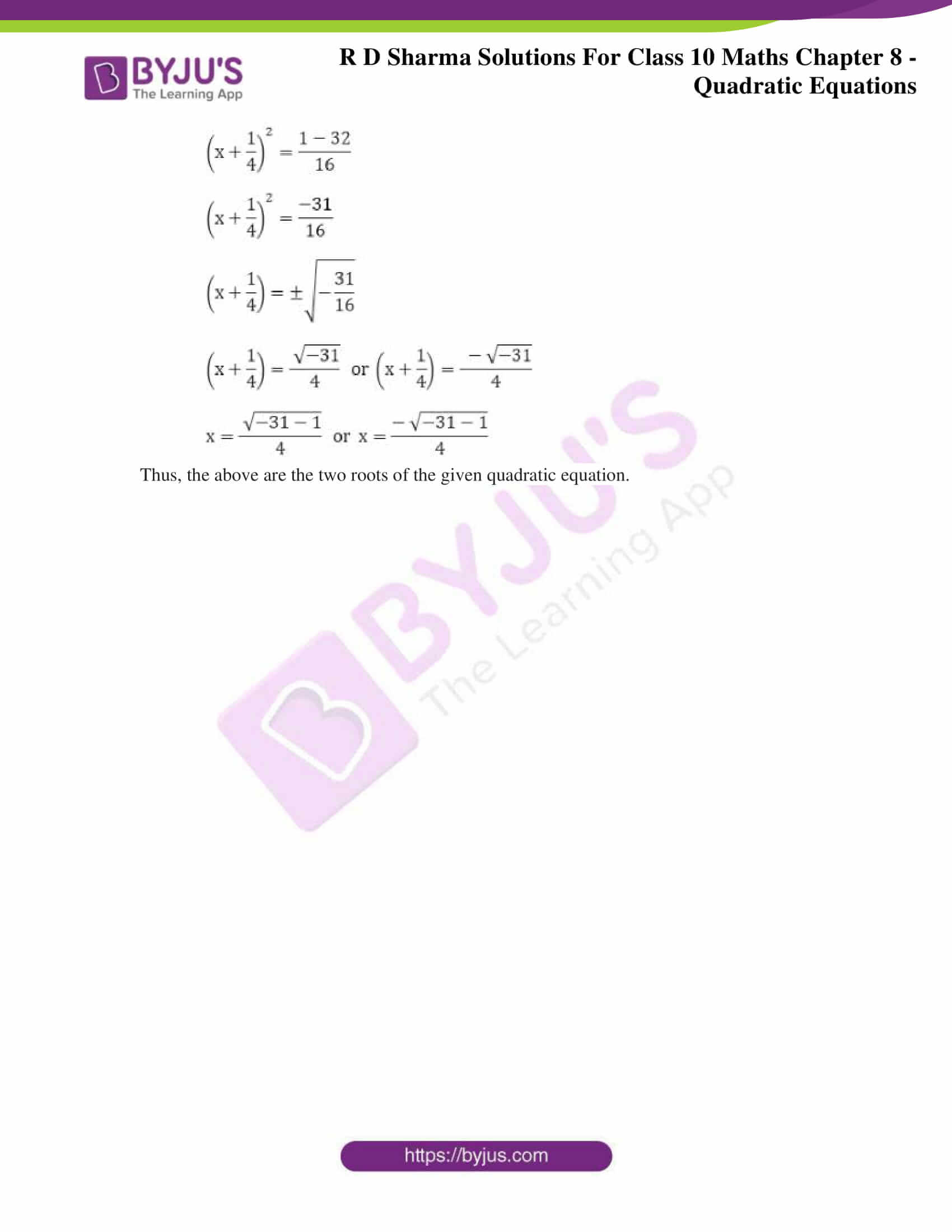### Access RD Sharma Solutions for Class 10 Maths Chapter 8 Quadratic Equations Exercise 8.4

Find the roots of the following quadratic equations (if they exist) by the method of completing the square.

1. x2 – 4√2 x + 6 = 0

Solution:

Given equation,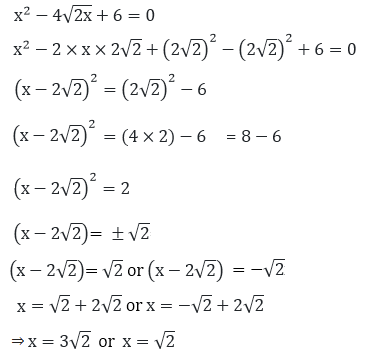Thus, the roots of the given quadratic equation are x = 3√2 and x = √2.

2. 2x2 – 7x + 3 = 0

Solution:

Given equation,

2x2 – 7x + 3 = 0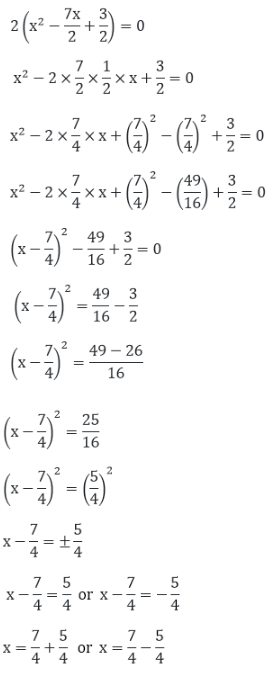⇒ x = 12/4 = 3 or x = 2/4 = 1/2

Thus, the roots of the given quadratic equation are x = 3 and x = 1/2.

3. 3x2 + 11x + 10 = 0

Solution:

Given equation,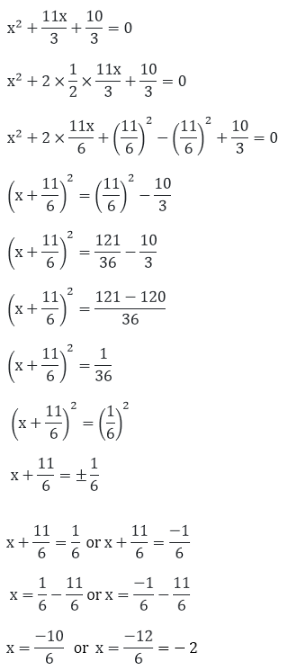⇒ x = -5/3 = 3 or x = -2

Thus, the roots of the given quadratic equation are x = -5/3 and x = -2.

4. 2x2 + x – 4 = 0

Solution:

Given equation,

2x2 + x – 4 = 0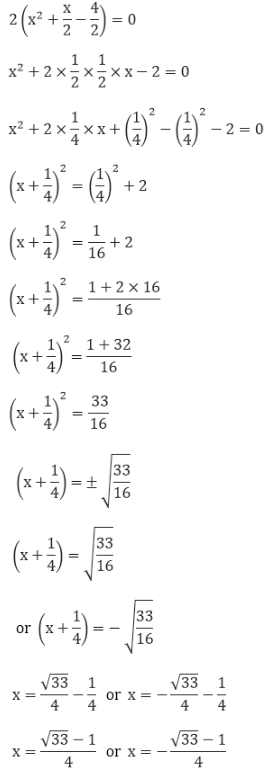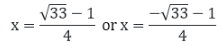Thus, the roots of the given quadratic equation are

5. 2x2 + x + 4 = 0

Solution:

Given equation,

2x2 + x + 4 = 0

x2 + x/2 + 2 = 0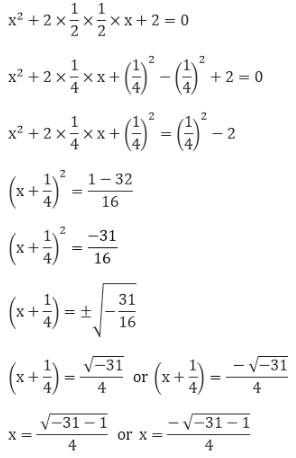Thus, the above are the two roots of the given quadratic equation.